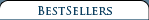3-View Scale Airplane Drawing Books & CDs Aero Albums Books Air Force Legends Air Racing Books Airliner Books Aviation CDs Aviation Century Series Biographicals British Aviation Books Children's Aviation Books Civil Aviation Books A-G Civil Aviation Books H-N Civil Aviation Books O-S Civil Aviation Books T-Z DVDs & Videos Early Aviation Books Golden Age Era Golden Age Of Aviation Series Military Aviation Modeling & R/C Airplane Books Naval Fighters Navy Books Nose Art Books Paul Matt Individual 3-View Drawings Personal Adventures Post World War II Books Soaring, Ultralights & Ballooning Time-Life's Epic Of Flight Series Type Airplane Books A-G Type Airplane Books H-P Type Airplane Books R-Z World War I Aviation Books World War II Aviation Books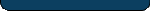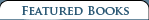Stinson's Golden Age - Vol 1 & Vol 2 \$ 50.00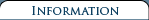Shipping and Returns   Terms and Conditions  Privacy Policy  Contact Us### Exploring the Monster - Mountain Lee Waves: The Aerial Elevator

 Retail Price: \$26.00 By: Robert F. Whelan Mountain lee waves and the rotors which so often accompany them comprise one of the most powerful forces in nature. Researchers, primarily flying sailplanes at record-setting altitudes, discovered ascent/descent rates exceeding 2,500 fpm, often in eerie calmness, and encountered the tremendous destructive power of rotors beneth these lee waves, capable of producing vertical/horizontal accelerations in excess of +/-15Gs.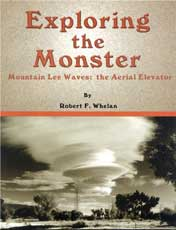These forces are more powerful than any private or commercial aircraft is designed to withstand. No pilot who flies in the lee of mountains can reasonably challenge a rotor, or ignore the effect of lee waves.

This is the fascinating account of the discovery and research into mountain lee waves and the rotors which so often accompany them. Said on reviewer, "This is a must read for every pilot."

Exploring the Monster is the story of the people and events, and the conclusions, of the Sierra Wave Project and the Jet Stream Project. Exploring the Monster is a fascinating and necessary read for everyone interested in aviation or anyone interested in learning more about a stunning-and common-force in nature.

Exploring the Monster - Mountain Lee Waves: The Aerial Elevator
Book Contents

ChapterВ В В В В В В В В В В В В В В В В В В В В В В В В В В В В В В В В В В В В В В В В В В В В В В В В В В В В В В В В В В В В В В В В В В В В В В В  Page

1В В В В В В В В В  Nature of the BeastВ В В В В В В В В В В В В В В В В В В В В В В В В В В В В В В В В В В В В В В В В В В В В В В В В В В В В В В В В В В В В В В В В В  1
2В В В В В В В В В  The West Coast WizardВ В В В В В В В В В В В В В В В В В В В В В В В В В В В В В В В В В В В В В В В В В В В В В  В В В В В В В В В В В  13
3В В В В В В В В В  Melting PotВ В В В В В В В В В В В В В В В В В В В В В В В В В В В В В В В В В В В В В В В В В В В В В В В В В В В В В В В В В В В В В В В В В В В В В В В В В В В В В  22
4В В В В В В В В В  BishopвЂ™s VisionaryВ В В В В В В В В В В В В В В В В В В В В В В В В В В В В В В В В В В В В В В В В В В В В В В В В В В В В В В В В В В В В В В В В В В  30
5В В В В В В В В В  Sierra Wave Project GliderВ В В В В В В В В В В В В В В В В В В В В В В В В В В В В В В В В В В В В В В В В В В В В В В В В В В В В В  39
6В В В В В В В В В  The Holiday ElevatorВ В В В В В В В В В В В В В В В В В В В В В В В В В В В В В В В В В В В В В В В В В В В В В В В В В В В В В В В В В В В В В В  44
7В В В В В В В В В  Birth of an IdeaВ В В В В В В В В В В В В В В В В В В В В В В В В В В В В В В В В В В В В В В В В В В В В В В В В В В В В В В В В В В В  61
8В В В В В В В В В  The Sierra Wave ProjectВ В В В В В В В В В В В В В В В В В В В В В В В В В В В В В В В В В В В В В В В В В В В В В В В В В В В В В В В В  69
9В В В В В В В В В  The Monster BitesВ В В В В В В В В В В В В В В В В В В В В В В В В В В В В В В В В В В В В В В В В В В В В В В В В В В В В В В В В В В В В В В В В В В  96
10В В В В В В В  Into the Jet StreamВ В В В В В В В В В В В В В В В В В В В В В В В В В В В В В В В В В В В В В В В В В В В В В В В В В В В В В В В В В В В В В В В В В В  110
11В В В В В В В  The Jet Stream ProjectВ В В В В В В В В В В В В В В В В В В В В В В В В В В В В В В В В В В В В В В В В В В В В В В В  125
12В В В В В В В  Aeolus Sends a SignalВ В В В В В В В В В В В В В В В В В В В В В В В В В В В В В В В В В В В В В В В В В В В В В В В В В В В В В В В В В В В В В  135
13В В В В В В В  AssessmentВ В В В В В В В В В В В В В В В В В В В В В В В В В В В В В В В В В В В В В В В В В В В В В В В В В В В В В В В В В В В В В В В В В В В В В В В В В В В В  144
EpilogueВ В В В В В В В В В В В В В В В В В В В В В В В В В В В В В В В В В В В В В В В В В В В В В В В В В В В В В В В В В В В В В В В В В В В В В В В В В В В В В В В В В В В В В В В В В В В В В  148

170 pages, softbound, 8ВЅ x 11, 74 photos.

### Exploring the Monster - Mountain Lee Waves: The Aerial Elevator

#### Item Number: 149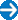Type in the title of book or similar words to help find your item(s).